### When gaming is NP-hard

by @ulaulaman about #candycrush #bejeweled #shariki #nphard #computerscienceShariki is a puzzle game developed by the russian programmer Eugene Alemzhin in 1994. The rules are simple:
(...) matching three or more balls of the same color in line (vertical or horizontal). These balls then explode and a new ones appear in their place.
The first Shariki's clone is Tetris Attack, a fusion between Shariki and the most famous Tetris, also this developed in Soviet Union by Alexey Pajitnov. But the most famous clone is Bejeweled (2001) by PopCap Games, from which is derived the Candy Crush Saga. During this March, Toby Walsh and the italian team composed by Luciano Gualà, Stefano Leucci, Emanuele Natale proved that Candy Crush and other similar games are NP-hard:
The twentieth century has seen the rise of a new type of video games targeted at a mass audience of "casual" gamers. Many of these games require the player to swap items in order to form matches of three and are collectively known as tile-matching match-three games. Among these, the most influential one is arguably Bejeweled in which the matched items (gems) pop and the above gems fall in their place. Bejeweled has been ported to many different platforms and influenced an incredible number of similar games. Very recently one of them, named Candy Crush Saga enjoyed a huge popularity and quickly went viral on social networks. We generalize this kind of games by only parameterizing the size of the board, while all the other elements (such as the rules or the number of gems) remain unchanged. Then, we prove that answering many natural questions regarding such games is actually NP-Hard. These questions include determining if the player can reach a certain score, play for a certain number of turns, and others.
The italian team realized also a web-based implementation of their technique.
Toby Walsh (2014). Candy Crush is NP-hard, arXiv:
Luciano Gualà, Stefano Leucci & Emanuele Natale (2014). Bejeweled, Candy Crush and other Match-Three Games are (NP-)Hard, arXiv:

### A blink of an eye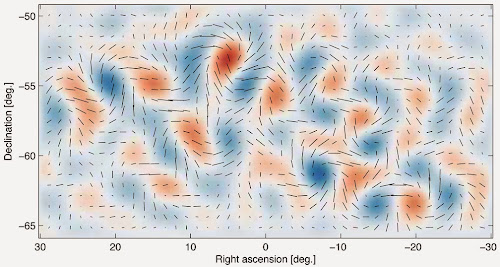Almost 14 billion years ago, the universe we inhabit burst into existence in an extraordinary event that initiated the Big Bang. In the first fleeting fraction of a second, the universe expanded exponentially, stretching far beyond the view of our best telescopes. All this, of course, was just theory.
Researchers from the BICEP2 collaboration today announced the first direct evidence for this cosmic inflation. Their data also represent the first images of gravitational waves, or ripples in space-time. These waves have been described as the "first tremors of the Big Bang." Finally, the data confirm a deep connection between quantum mechanics and general relativity.
(from the press release)
In physics, gravitational waves are ripples in the curvature of spacetime that propagate as a wave, travelling outward from the source. Predicted in 1916 by Albert Einstein to exist on the basis of his theory of general relativity, gravitational waves theoretically transport energy as gravitational radiation. Sources of detectable gravitational waves could possibly include binary star systems composed of white dwarfs, neutron stars, or black holes. The existence of gravitational waves is a possible consequence of the Lorentz invariance of general relativity since it brings the concept of a limiting speed of propagation of the physical interactions with it. Gravitational waves cannot exist in the Newtonian theory of gravitation, in which physical interactions propagate at infinite speed.
(from Wikipedia) Cosmic inflation was introduced by Alan Guth and Andrei Linde in 1981:
One of the intriguing consequences of inflation is that quantum fluctuations in the early universe can be stretched to astronomical proportions, providing the seeds for the large scale structure of the universe. The predicted spectrum of these fluctuations was calculated by Guth and others in 1982. These fluctuations can be seen today as ripples in the cosmic background radiation, but the amplitude of these faint ripples is only about one part in 100,000. Nonetheless, these ripples were detected by the COBE satellite in 1992, and they have now been measured to much higher precision by the WMAP satellite and other experiments. The properties of the radiation are found to be in excellent agreement with the predictions of the simplest models of inflation.
(from MIT)
So our universe is born after a quantum blink of an eye, or like a solution of the equation of everything.
In the following a storify with a collection of link about one of the most important discovery of the... universe!

### A brief history of pi: part 2

by @ulaulaman about #piday #pi #MachinFormula #EulerIdentity
Today is the pi day, so I continue the brief history of $\pi$
After the introduction of $\pi$ in mathematics, one of the quest linked with the calculation of its digits is the research about its nature, or in other words what kind of number it is. Numbers classification is simple for all: we start with natural numbers (positive and negative), and so we can define rational numbers, as the numbers generated by the ratio between two natural numbers. Every rational number could be expressed like $\frac{a}{b}$, with $a$, $b$ natural and $b$ not null.
Johann Heinrich Lambert was the first to show the irrational nature of $\pi$ in 1761 in Mémoire sur quelques propriétés remarquables des quantités transcendantes circulaires et logarithmiques: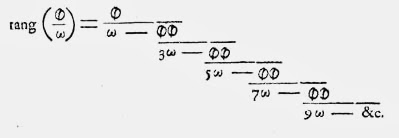commons
that could be written in this way: $\tan(x) = \cfrac{x}{1 - \cfrac{x^2}{3 - \cfrac{x^2}{5 - \cfrac{x^2}{7 - {}\ddots}}}}$ Lamberd proved that if $x$ is not null and rational, then the previous expression must be irrational. So the irrationality of $\pi$ follows from $\tan (\pi /4) = 1$. A good synthesis of Lambert's proof is on The world of $\pi$.
In 1997 Laczkovich proposed a simplification of this demonstration, while another variation was proposed in 2009 by Li Zhou, using the integral calculus. In particular the second demonstration is inspired by the proof that Charles Hermite written in two letters to Paul Gordan and Carl Borchardt in 1873. Following Harold Jeffreys in Scinetific interference (1973), a simplification of this proof, that used a reductio ab adsurdum is proposed by Mary Cartwright.
Another proof of one page about the irrationality of $\pi$ is dued by Ivan Niven in 1946.
At the other hand, the transcendence of $\pi$ is a direct consequence of the Lindemann-Weierstrass theorem:
If $\alpha_1$, $\cdots$, $\alpha_n$ are algebraic numbers that are linearly independent over rationals, then $e^{\alpha_1}$, $\cdots$, $e^{\alpha_n}$ are algebraically independent over rationals.
where an algebraic number is the solution of a polynomial equation with rational coefficients.
In 1882 Lindemann, using this theorem, showed that $e$ is transcendental, and, like a consequence of the Euler's identity, also $\pi$ is transcendental.

### Stephen Hawking and the (cosmological) Riemann's zeta function

Following Emilio Elizalde (read this presentation in pdf) I found a paper by Stephen Hawking in which he used the Riemann's zeta function:
This paper describes a technique for regularizing quadratic path integrals on a curved background spacetime. One forms a generalized zeta function from the eigenvalues of the differential operator that appears in the action integral. The zeta function is a meromorphic function and its gradient at the origin is defined to be the determinant of the operator. This technique agrees with dimensional regularization where one generalises ton dimensions by adding extra flat dimensions. The generalized zeta function can be expressed as a Mellin transform of the kernel of the heat equation which describes diffusion over the four dimensional spacetime manifold in a fith dimension of parameter time. Using the asymptotic expansion for the heat kernel, one can deduce the behaviour of the path integral under scale transformations of the background metric. This suggests that there may be a natural cut off in the integral over all black hole background metrics. By functionally differentiating the path integral one obtains an energy momentum tensor which is finite even on the horizon of a black hole. This energy momentum tensor has an anomalous trace.
Hawking used the following version for the zeta: $\zeta (s) = \sum_n \lambda_n^{-s}$ where $\lambda_n$ are the eigenvalues of a given operator $A$, constructed using the background fields of the spacetime. In four dimensions the function will converge for $\Re (s) > 2$.
About the use of the Riemann's zeta function in physics, you could also read Effective Lagrangian and energy-momentum tensor in de Sitter space
Hawking S.W. (1977). Zeta function regularization of path integrals in curved spacetime, Communications in Mathematical Physics, 55 (2) 133-148. DOI: (pdf)

### Extracting energy from a black hole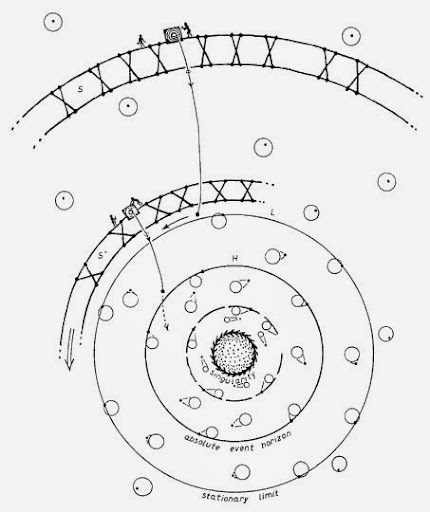The Penrose process is a process theorised by Roger Penrose wherein energy can be extracted from a rotating black hole. That extraction is made possible because the rotational energy of the black hole is located, not inside the event horizon of the black hole, but on the outside of it in a region of the Kerr spacetime called the ergosphere, a region in which a particle is necessarily propelled in locomotive concurrence with the rotating spacetime. All objects in the ergosphere become dragged by a rotating spacetime. In the process, a lump of matter enters into the ergosphere of the black hole, and once it enters the ergosphere, it is split into two. The momentum of the two pieces of matter can be arranged so that one piece escapes to infinity, whilst the other falls past the outer event horizon into the hole. The escaping piece of matter can possibly have greater mass-energy than the original infalling piece of matter, whereas the infalling piece has negative mass-energy. In summary, the process results in a decrease in the angular momentum of the black hole, and that reduction corresponds to a transference of energy whereby the momentum lost is converted to energy extracted.
The process obeys the laws of black hole mechanics. A consequence of these laws is that if the process is performed repeatedly, the black hole can eventually lose all of its angular momentum, becoming non-rotating, i.e. a Schwarzschild black hole. Demetrios Christodoulou calculated an upper bound for the amount of energy that can be extracted by the Penrose process.
And Reva-Kay Williams used the Penrose process to explain the collimated and asymmetrycal jets from some space objects, like rotating black holes:
Over the past three decays, since the discovery of quasars, mounting observational evidence has accumulated that black holes indeed exist in nature. In this paper, I present a theoretical and numerical (Monte Carlo) fully relativistic 4-D analysis of Penrose scattering processes (Compton and $\gamma \gamma \rightarrow e^+ e^-$) in the ergosphere of a supermassive Kerr (rotating) black hole. These model calculations surprisingly reveal that the observed high energies and luminosities of quasars and other AGNs, the collimated jets about the polar axis, and the asymmetrical jets (which can be enhanced by relativistic Doppler beaming effects), all, are inherent properties of rotating black holes. That is, from this analysis, it is shown that the Penrose scattered escaping particles exhibit tightly wounded coil-like cone distributions (highly collimated jet distributions) about the polar axis, with helical polar angles of escape varying from 0.5o to 30o for the highest energy particles. It is also shown that the gravitomagnetic (GM) field, which causes the dragging of inertial frames, exerts a force acting on the momentum vectors of the incident and scattered particles, causing the particle emission to be asymmetrical above and below the equatorial plane, thus breaking the reflection symmetry of the Kerr metric (above and below the equatorial plane). When the accretion disk is assumed to be a two-temperature bistable thin disk/ion corona, recently referred to as an advection dominated accretion flow (ADAF), energies as high as 54 GeV can be attained by these Penrose processes alone; and when relativistic beaming is included, energies in the TeV range can be achieved, agreeing with observations of some BL Lac objects. When this model is applied specifically to quasars 3C 279 and 3C 273, their observed high energy luminosity spectra can be duplicated and explained. Moreover, this Penrose energy extraction model can be applied to any size black hole, irrespective of the mass, and, thus, suggests a complete theory for the extraction of energy from a black hole.

Williams, R.K., High Energy-Momentum Extraction from Rotating Black Holes Using the Penrose Mechanism. American Astronomical Society, 195th AAS Meeting, #134.02; Bulletin of the American Astronomical Society, Vol. 32, p.881
Reva Kay Williams (2002). The Gravitomagnetic Field and Penrose Processes, arXiv:
Penrose, R., Gravitational Collapse: the Role of General Relativity. Rivista del Nuovo Cimento, Numero Speziale I, 252 (1969) (pdf)

### Bikini

#Bikini #BikiniAtoll #fallout #ColdWarThe United States was in a Cold War Nuclear arms race with the Soviet Union to build bigger and better bombs. The next series of tests over Bikini Atoll was code named Operation Castle. The first test of that series was Castle Bravo, a new design utilizing a dry fuel thermonuclear hydrogen bomb. It was detonated at dawn on March 1, 1954.
The 15 megaton nuclear explosion far exceeded the expected yield of 4 to 8 megatons (6Mt predicted), and was about 1,000 times more powerful than each of the atomic bombs dropped on Hiroshima and Nagasaki during World War II. The nuclear weapon was the most powerful device ever detonated by the United States (and just under one-third the energy of the Tsar Bomba, the largest ever tested). The scientists and military authorities were shocked by the size of the explosion and many of the instruments they had put in place to evaluate the effectiveness of the device were destroyed.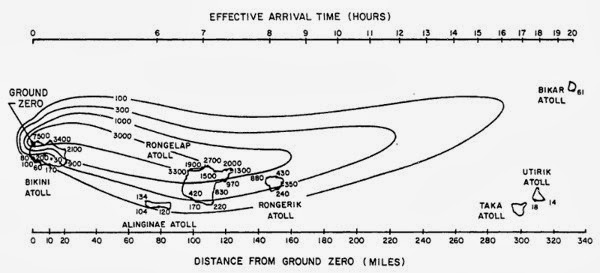The Bravo fallout plume spread dangerous levels of radiation over an area over 100 miles (160 km) long, including inhabited islands. The contour lines show the cumulative radiation dose in roentgens (R) for the first 96 hours after the test.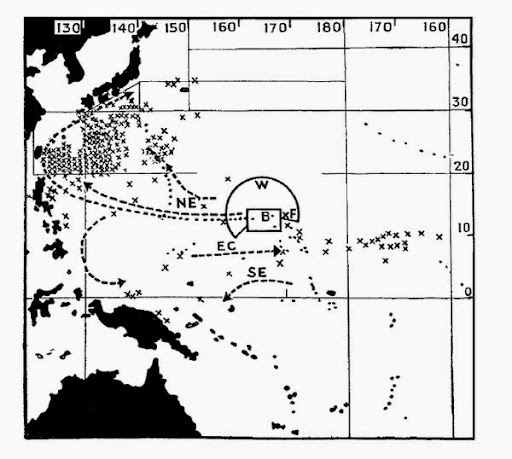Map showing points (X) where contaminated fish were caught or where the sea was found to be excessively radioactive.
B = original "danger zone" around Bikini announced by the U.S. government.
W = "danger zone" extended later.
xF = position of the Lucky Dragon fishing boat.
NE, EC, and SE are equatorial currents.

Read also: A Short History of the People of Bikini Atoll
(source: Wikipedia)